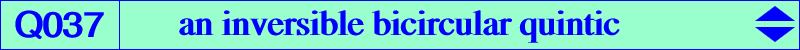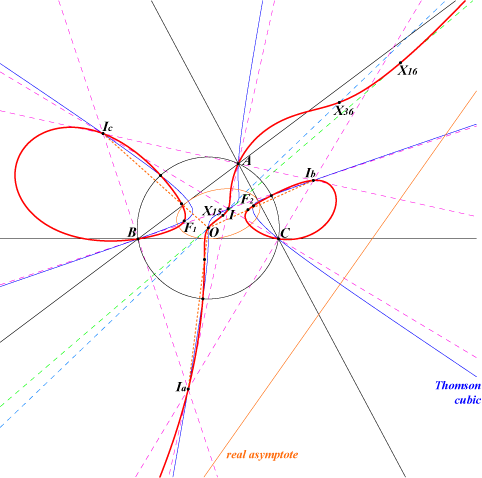too complicated to be written here. Click on the link to download a text file.X(1), X(3), X(15), X(16), X(30), X(36), X(5000), X(5001) Ia, Ib, Ic : excenters extraversions of X(36) foci of the Steiner inscribed ellipse anti-points, see Table 77 vertices of the Thomson triangle i.e. common points of the circumcircle and the Thomson cubic X(5)-circumcevian points (see Q141)Q037 is the isogonal transform of Q030. It is an inversible bicircular quintic with a real asymptote parallel to the Euler line. The tangents at A, B, C, X(15), X(16) and the vertices of the Thomson triangle concur at O hence Q037 is bitangent at X(15), X(16) to the Brocard axis. More generally, the polar line of M in Q037 passes through O if and only if M lies on Q136. In other words, Q136 is the 4th polar of O in Q037. The 1st polar is the Euler line, the 2nd polar is the Stammler hyperbola, the 3rd polar is a cubic passing through O, X(371), X372). The tangents at O, X(1), Ia, Ib, Ic concur at H. The Laplacian of Q037 is K463. This is the locus of M whose polar conic in Q037 is a rectangular hyperbola. Q037 is the locus of P such that the Euler line of the circumcevian triangle of P contains P (and obviously O). See a generalization at Q067. See also the similar quintic Q054 and Table 55. See another property here (Angel Montesdeoca, 2020-08-17).